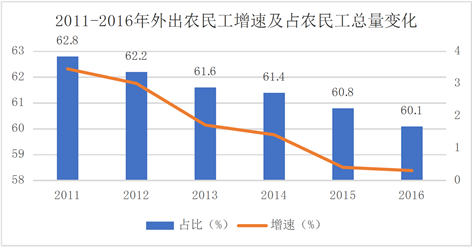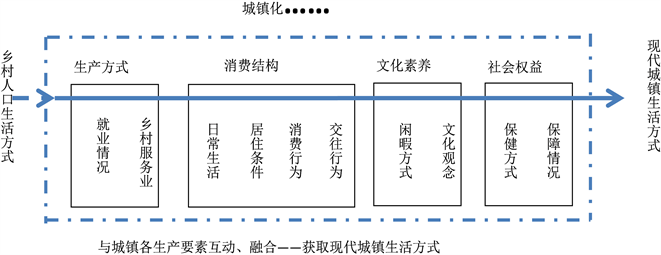﻿ 基于生活方式城市性的浙江乡村人口就地城镇化测评

基于生活方式城市性的浙江乡村人口就地城镇化测评Evaluation of Zhejiang Rural Population Local Urbanization Based on the Urbanity of Lifestyle

Abstract: The rural urbanization in China is the mainly factor of improving the quality of the China’s mod-ernization, while the rural urbanization is the local and near urbanization. According to the new urbanization and the Rural Revitalization strategy, the essence of the rural local urbanization is the life-style urbanization. And the studies on local urbanization mostly concentrated on qualitative research. So this paper constructs an index system to measure the urbanization level and quality of rural local population urbanization based on the urbanity of lifestyle, and by the improved entropy method to evaluate the level and quality in Zhejiang from 2010 to 2016. Finally, we analyze the coupling and coordination relationship between rural local urbanization level and quality in three major economic zones, namely, Shanghai-Hangzhou half-hour economic zone, coastal economic zone and Western Zhejiang inland zone. The results show that the coupling and coordination of each region is in the ascending stage, but in the spatial agglomeration the development level and quality show a decreasing trend of coastal economic zone, Shanghai-Hangzhou half-hour economic zone and Western Zhejiang inland area; although the geographical factors have an important impact on it, the government system and the way of economic development are the decisive factors.

1. 引言

2. 乡村人口就地城镇化测评指标体系构建

2.1. 乡村人口就地城镇化概定与测评理论依据数据来源：《2016年农民工监测调查报告》

Figure 1. Changes in the growth rate of migrant workers and the proportion of migrant workers in 2011~2016Figure 2. Schematic diagram of the process of urbanization of rural population in urbanization

2.2. 乡村人口就地城镇化测评指标选取Table 1. Urban population lifestyle evaluation index system

3. 数据处理、分析方法与计算结果

3.1. 乡村人口生活方式城镇化水平计算

$乡村人口生活方式城镇化率=\frac{乡村私营企业就业人数+乡村个体就业人数}{乡村人口数}$ (1)

Table 2. Urbanization level of rural population in Zhejiang Province (%)

3.2. 乡村人口生活方式城镇化质量测度

3.2.1. 改进功效函数

${x}_{ij}^{*}=\left\{\begin{array}{l}\alpha ×\frac{{X}_{ij}-{x}_{ij\mathrm{min}}}{{x}_{ij\mathrm{max}}-{x}_{ij\mathrm{min}}}+\left(1-\alpha \right)\text{\hspace{0.17em}}\text{\hspace{0.17em}}正功效\text{\hspace{0.17em}}\text{\hspace{0.17em}}\left(i=1,2,\cdots ,m;j=1,2,\cdots ,n\right)\\ \alpha ×\frac{{x}_{ij\mathrm{max}}-{X}_{ij}}{{x}_{ij\mathrm{max}}-{x}_{ij\mathrm{min}}}+\left(1-\alpha \right)\text{\hspace{0.17em}}\text{\hspace{0.17em}}负功效\text{\hspace{0.17em}}\text{\hspace{0.17em}}\left(i=1,2,\cdots ,m;j=1,2,\cdots ,n\right)\end{array}$ (2)

3.2.2. 熵值法赋权

${p}_{ij}=\frac{{y}_{ij}}{{\sum }_{i=1}^{n}{y}_{ij}}\text{\hspace{0.17em}}\text{\hspace{0.17em}}\left(i=1,2,\cdots ,n\right)$

${e}_{j}=\frac{1}{\mathrm{ln}\left(m\right)}\underset{j=1}{\overset{n}{\sum }}{p}_{ij}\text{\hspace{0.17em}}\text{\hspace{0.17em}}\left(j=1,2,\cdots ,n\right)$

${w}_{j}=\frac{1-{e}_{j}}{{\sum }_{j=1}^{n}\left(1-{e}_{j}\right)}$

3.2.3. 方案得分与质量测度Table 3. Urbanization quality of rural population in Zhejiang Province

3.3. 耦合协调性测度

${C}_{n}={\left\{\left({u}_{1}×{u}_{2}×\cdots ×{u}_{n}\right)/\left[{\prod }^{\text{​}}\left({u}_{i}+{u}_{j}\right)\right]\right\}}^{\frac{1}{n}}$

$D={\left(C×T\right)}^{\frac{1}{2}}$ , $T=\alpha ×{U}_{1}+\beta ×{U}_{2}$

Table 4. Coupling and coordination level of urbanization and lifestyle of rural population in Zhejiang Province from 2010 to 2016

4. 结果分析

4.1. 发展水平总体呈扬抑交错，政策推力是其发展主动力Figure 3. Urbanization level of rural population lifestyle in Zhejiang’s three major regional economic belts

4.2. 发展质量稳步提升，区域间协同明显Figure 4. Urbanization quality of rural population lifestyle in Zhejiang’s three major regional economic belts

4.3. 耦合协调度时序上逐年提升，空间集聚效应显著Table 5. Coupling degree and coupling coordination degree

5. 结论与讨论

2018年国家社科基金重大项目(18ZDA093)；2016年度浙江省哲学社会科学重点研究基地(浙江城市治理研究中心)重点项目(16jdgh002)：浙江省就地城镇化发展水平与质量协调发展研究。

 李强, 王昱. 什么是人的城镇化[J]. 南京农业大学学报, 2017, 17(2): 1-7.

 李健, 杨传开, 宁越敏. 新型城镇化背景下的就地城镇化发展机制与路径[J]. 学术月刊, 2016(7): 89-97.

 吴业苗. 农业转移人口的新常态与市民化进路[J]. 农业经济问题, 2016(3): 43-50.

 黄璜, 杨贵庆, 菲利普米赛尔维茨, 等. “后乡村城镇化”与乡村振兴——当代德国乡村规划探索及对中国的启示[J]. 城市规划, 2017(11): 111-119.

 王景新. 中国农村发展新阶段:村域城镇化[J]. 中国农村经济, 2015(10): 4-14.

 王业强, 魏后凯. 大城市效率锁定与中国城镇化路径选择[J]. 中国人口科学, 2018(2): 24-38.

 薛德升, 曾献君. 中国人口城镇化质量评价及省级差异分析[J]. 地理学报, 2016(2): 194-204.

 张文忠. 新型城镇化的核心是生活方式的转变[J]. 北京规划建设, 2014(6): 47-51.

 李强. 人的城镇化本意[J]. 理论与当代, 2018(7): 59.

 朱宇, 祁新华, 王国栋. 中国就地城镇化: 理论与实践[M]. 北京: 科学出版社, 2012.

 李强, 赵罗英. 中国中等收入群体和中等生活水平研究[J]. 河北学刊, 2017(5): 151-157.

 胡滨. 人口城市化、空间城市化与社会风险——对我国当代城市化的一项剖析[J]. 社会科学, 2012(5): 59-68.

 Ma, L., Jin, F., Song, Z., et al. (2013) Spatial Coupling Analysis of Regional Economic Development and En-vironmental Pollution in China. Journal of Geographical Sciences, 23, 525-537.

 刘耀彬, 宋学锋. 城市化与生态环境的耦合度及其预测模型研究[J]. 中国矿业大学学报, 2005(1): 91-96.

Top